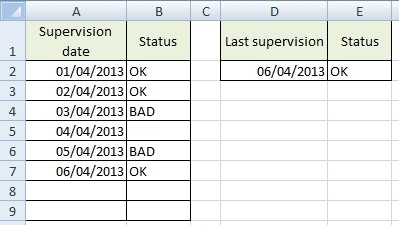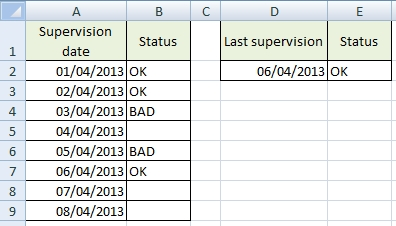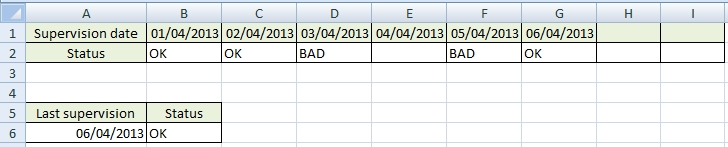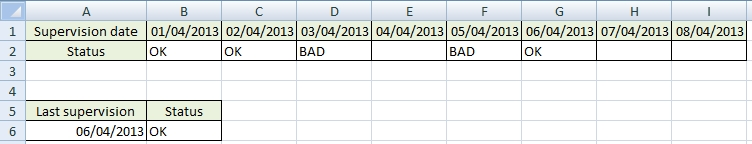# Value of the last filled cell in a column or in a row

I’m using Array Formulas to find the last filled or the last non empty cell ,it’s the same :). Important: Each formula must be confirmed by Ctrl+Shift+Enter (not just Enter).

## Example 1 / Last filled cell in a column

This formulas are for the entire column.

Formula from cell A1 to A12
=INDEX(A1:A12,MAX((A1:A12<>””)*(ROW(A1:A12))))

Cell A1 is fixed and A12 is optional. If you need another column, just change letter A in the formula. The advantage of this formula is that it works in all Excel versions. The disadvantage is that the searched range must start in the first row (A1, B1, C1, …).

Formula for column A
=INDEX(A:A,MAX((A:A<>””)*(ROW(A:A))))

This works only in Excel 2007 and higher (Excel 2003 displays #NUM! error).

### Formula for the table column / example 1

From a practical point of view is not very convenient to search the entire Excel column.We want to find the last filled cell in the first and the second column of the table.

Formula for Last supervision (cell D2)
=OFFSET(A2,MAX((A2:A9<>””)*(ROW(A2:A9)))-ROW(A2),0)

If you see some number instead of the date, just change the cell format to Date.

Formula for the last Status (cell E2)
=OFFSET(B2,MAX((B2:B9<>””)*(ROW(B2:B9)))-ROW(B2),0)

This works for all Excel versions. I note that it is necessary to confirm the formula with Ctrl + Shift + Enter.

### Formula for the table column / example 2

The table contains prefilled values in the first column. We want to find the last record having Status.Formula for Last supervision (cell D2)
=OFFSET(A2,MAX((B2:B9<>””)*(ROW(B2:B9)))-ROW(B2),0)

Formula for the last Status (cell E2)
=OFFSET(B2,MAX((B2:B9<>””)*(ROW(B2:B9)))-ROW(B2),0)

## Example 2 / Last filled cell in a row

This formulas are for the entire row.

Formula from cell A1 to Z1
=INDEX(A1:Z1,0,MAX((A1:Z1<>””)*(COLUMN(A1:Z1))))

Cell A1 is fixed and Z1 is optional. If you need another row, just change number 1 in the formula. The advantage of this formula is that it works in all Excel versions. The disadvantage is that the searched range must start in the first column (A1, A2, A3, …).

Formula for row 1
=INDEX(1:1,0,MAX((1:1<>””)*(COLUMN(1:1))))

This works only in Excel 2007 and higher (Excel 2003 displays #NUM! error).

### Formula for the table row / example 1

We want to find the last filled cell in the first and the second row of the table.Formula for Last supervision (cell A6)
=OFFSET(B1,0,MAX((B1:I1<>””)*(COLUMN(B1:I1)))-COLUMN(B1))

If you see some number instead of the date, just change the cell format to Date.

Formula for the last Status (cell B6)
=OFFSET(B2,0,MAX((B2:I2<>””)*(COLUMN(B2:I2)))-COLUMN(B2))

Note: it is necessary to confirm the formulas with Ctrl + Shift + Enter (array formula).

### Formula for the table row / example 2

The table contains prefilled values in the first row. We want to find the last record having Status.Formula for Last supervision (cell A6)
=OFFSET(B1,0,MAX((B2:I2<>””)*(COLUMN(B2:I2)))-COLUMN(B2))

Formula for the last Status (cell B6)
=OFFSET(B2,MAX((B2:B9<>””)*(ROW(B2:B9)))-ROW(B2),0)

1.This solved my issue with array formula. Thank you

2.Nice formulas

3.Hi.
Nice post. I have a further issue. Talking about Formula for the table row / example 1 and 2, suppose we are looking for a non standard/fixed value (loke “OK” or “BAD”) but we need to find a number that could be also repeteable (like, 1,3,2,3,4,5, ,6,2,,, and so on) how I can it?
If it is not clear you could just replave the last OK and BAD with some numbers and find last cell was filled in the row.